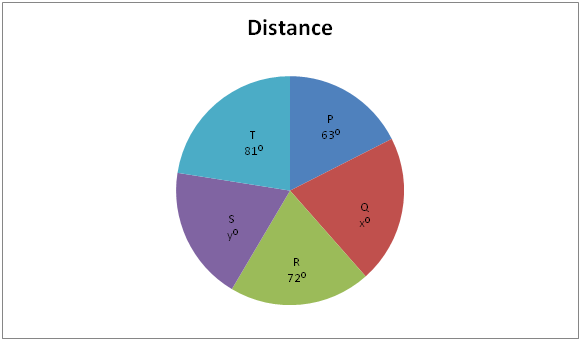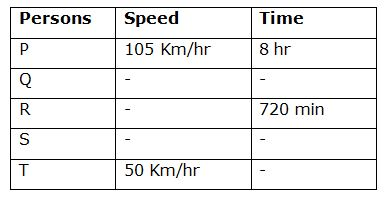# SBI Clerk/ IDBI and South Indian Bank Mains Quantitative Aptitude Questions 2019 (Day-04)

Dear Aspirants, Our IBPS Guide team is providing a new series of Quantitative Aptitude Questions for SBI Clerk /IDBI and South Indian Bank Mains 2019 so the aspirants can practice it on a daily basis. These questions are framed by our skilled experts after understanding your needs thoroughly. Aspirants can practice these new series questions daily to familiarize with the exact exam pattern and make your preparation effective.

Check here for IBPS RRB Clerk Prelims Mock Test 2019

Check here for EPFO Assistant Mock Test 2019

Check here for LIC ADO Mock Test 2019

### Click Here to Subscribe Crack High Level Puzzles & Seating Arrangement Questions PDF 2019 Plan

[WpProQuiz 6729]

Directions (Q. 1 – 5): Following question contains two equations as I and II. You have to solve both equations and determine the relationship between them and give answer as,

a) x > y

b) x ≥ y

c) x = y or relationship can’t be determined.

d) x < y

e) x ≤ y

1) I) 5x + 4y – 20xy = 1

II) 8 + 6xy – 6x – 8y = 0

2) I) 5x2 – 20x + 15 = 0

II) y + ∛125 = 0.9

3) I) x2 + 73/2 = (71/2 + 7) x

II) y13 – (3439/2 / √y) = 0

4) I) 13x3 + 142x2 + 216x = 5x3 + 228x2

II) 5y2 – 21y + 18 = 0

5) I) 8x2 – (8 + 4√3) x + 4√3 = 0

II) 12y2 – (27 + 4√3) y + 9√3 = 0

Directions (Q. 6 – 10): Study the following information carefully and answer the questions given below.

The following pie chart shows the distance covered by 5 different persons. (In degrees)The table below shows the speed and time taken by the person to cover the given distance. Some values are missing here.6) If the time taken by Q is twice the time taken by P to cover the given distance and the ratio between the distance covered by P to that of Q is        5 : 6, then find the speed of Q?

a) 63 Km/hr

b) 57 Km/hr

c) 68 Km/hr

d) 52 Km/hr

e) None of these

7) With the help of question 6, find the average speed of P and Q?

a) 93 Km/hr

b) 65 Km/hr

c) 77 Km/hr

d) 84 Km/hr

e) None of these

8) If the distance covered by S is 912 km and the time taken by Q to cover the given distance is 12 hours, then find the ratio between the speeds of Q to that of R?

a) 29 : 15

b) 15 : 13

c) 23 : 19

d) 21 : 20

e) None of these

9) Find the total time taken by Q and T to cover the given distance, if the speed of Q is 65 Km/hr and the distance covered by S is 880 Km?

a) 42 hr 28 min

b) 37 hr 36 min

c) 35 hr 24 min

d) 40 hr 40 min

e) None of these

10) Find the sum of the average distance covered by P, R and T together and the average distance covered by Q and S together?

a) 1920 Km

b) 1840 Km

c) 1720 Km

d) 2160 Km

e) None of these

Direction (1-5) :

I) 5x + 4y – 20xy – 1 = 0

Divide by 20,

(5x/20) + (4y/20) – (20xy/20) – (1/20) = 0

(x/4) + (y/5) – xy – (1/20) = 0

xy – (x/4) – (y/5) + (1/20) = 0

x (x – ¼) – 1/5 (y – 1/4) = 0

(x – 1/5) (y – ¼) = 0

x = 1/5, y = ¼

II) 8 + 6xy – 6x – 8y = 0

Divide by 6,

(8/6) + (6xy/6) – (6x/6) – (8y/6) = 0

(4/3) + xy – x – (4y/3) = 0

X (y – 1) – (4/3) (y – 1) = 0

(x – 4/3) (y – 1) = 0

x = 4/3, y = 1

Can’t be determined

I) 5x2 – 20x + 15 = 0

5x2 – 5x – 15x + 15 = 0

5x (x – 1) – 15 (x – 1) = 0

(5x – 15) (x – 1) = 0

x = 3, 1

II) y + ∛125 = 0.9

y + 0.5 = 0.9

y = 0.9 – 0.5 = 0.4

x > y

I) x2 + 73/2 = (71/2 + 7) x

x2 – (√7 + 7) x + 7√7 = 0

x = 7, √7

II) y13 – (3439/2 / √y) = 0

[(y13 * y1/2) – (73)9/2] / √y = 0

y27/2 – 727/2 = 0

y27/2 = 727/2

y = 7

x ≤ y

I) 13x3 + 142x2 + 216x = 5x3 + 228x2

8x3 – 86x2 + 216x = 0

x (8x2 – 86x + 216) = 0

x [8x2 – 32x – 54x + 216] = 0

x [8x (x – 4) – 54 (x – 4)] = 0

x [(8x – 54) (x – 4)] = 0

x = 0, 54/8, 4

x = 0, 6.75, 4

II) 5y2 – 21y + 18 = 0

5y2 – 15y – 6y – 12 = 0

5y (y – 3) – 6 (y – 3) = 0

(5y – 6) (y – 3) = 0

y = 6/5, 3 = 1.2, 3

Can’t be determined

I) 8x2 – (8 + 4√3) x + 2√3 = 0

x = 8/8, 4√3/8

x = 0.866, 1

II) 12y2 – (27 + 4√3) y + 9√3 = 0

y = 27/12, 4√3/12

y = 2.25, 0.577

Can’t be determined

Direction (6-10) :

The distance covered by P = 105 * 8 = 840 Km

The time taken by Q = 2 * the time taken by P = 2 * 8 = 16 hr

The distance covered by Q = (840/5) * 6 = 1008 Km

Speed of Q = Distance/Time = 1008/16 = 63 Km/hr

The speed of P = 105 Km/hr

The distance covered by P = 105 * 8 = 840 Km

The time taken by Q = 2 * the time taken by P = 2 * 8 = 16 hr

The distance covered by Q = (840/5) * 6 = 1008 Km

Speed of Q = Distance/Time = 1008/16 = 63 Km/hr

Average Speed = Total distance/Total time

= > (840 + 1008) / (8 + 16)

= > 1848/24 = 77 Km/hr

The distance covered by P = 105*8 = 840 Km

630 = 840 km

30 = 40 km

10 = (40/3) Km

Total degree for circle = 3600

X0 + y0 = 360 – (63 + 72 + 81) = 1440

The distance covered by S = 912 km = y0

Y0 = (912/40)*3 = 68.40

The distance covered by Q = x0 = 144 – 68.40 = 75.60

The distance covered by Q = 75.6*(40/3) = 1008 Km

The time taken by Q to cover the given distance = 12 hours

The speed of Q = 1008/12 = 84 Km

The distance covered by R = 720 = 72*(40/3) = 960 Km

The speed of R = (960/12) = 80 Km/hr

Required ratio = 84 : 80 = 21 : 20

The distance covered by P = 105*8 = 840 Km

630 = 840 km

30 = 40 km

10 = (40/3) Km

Total degree for circle = 3600

x0 + y0 = 360 – (63 + 72 + 81) = 1440

The distance covered by S = 880 km = y0

y0 = (880/40) * 3 = 660

The distance covered by Q = x0 = 144 – 660 = 780 = 78*(40/3) = 1040 Km

The speed of Q = 65 Km/hr

The time taken by Q to cover the distance

= > (1040/65) = 16 hr

The distance covered by T = 810 = 81*(40/3) = 1080 Km

The time taken by T to cover the distance

= > (1080/50) = 21.6 hr (or) 21 hr 36 min

Required time = 16 + 21 hr 36 min = 37 hr 36 min

The distance covered by P = 105*8 = 840 Km

630 = 840 km

30 = 40 km

10 = (40/3) Km

The total distance covered by P, R and T together

= > 216 * (40/3) = 2880 Km

The average distance covered by P, R and T together

= > 2880/3 = 960 Km

Total degree for circle = 3600

x0 + y0 = 360 – (63 + 72 + 81) = 1440

The total distance covered by Q and S together = 144*(40/3) = 1920 Km

The average distance covered by Q and S together

= > 1920/2 = 960 Km

Required difference = 960 + 960 = 1920 Km